# Survival data analysis

Survival Analysis typically focuses on time to event data. In the most general sense, it consists of techniques for positive-valued random variables, such as

• time to death
• time to onset (or relapse) of a disease
• length of a contract
• duration of a policy
• money paid by health insurance
• viral load measurements
• time to finishing a master thesis

Typically, survival data are not fully observed, but rather are censored.

library(ggplot2)
library(survival)
library(KMsurv)


## Definitions and notation

Failure time random variables are always non-negative. That is, if we denote the failure time by $$T$$, then $$T\geq 0$$.

A random variable X is called a censored failure time random variable if $$X = \min(T,U)$$, where $$U$$ is a non-negative censoring variable.

In order to define a failure time random variable, we need:

1. an unambiguous time origin (e.g. randomization to clinical trial, purchase of car)
2. a time scale (e.g. real time (days, years), mileage of a car)
3. definition of the event (e.g. death, need a new car transmission)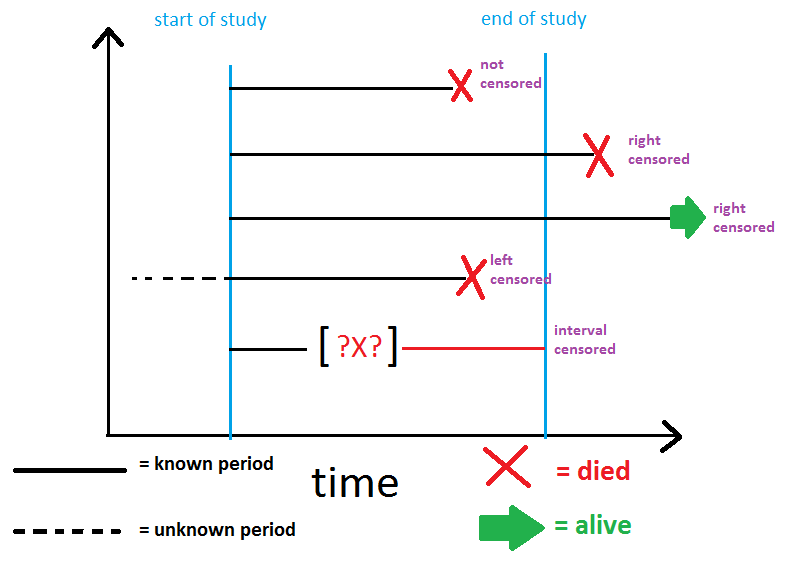The illustration of survival data shows several features which are typically encountered in analysis of survival data:

• individuals do not all enter the study at the same time
• when the study ends, some individuals still haven't had the event yet
• other individuals drop out or get lost in the middle of the study, and all we know about them is the last time they were still 'free' of the event

The first feature is referred to as 'staggered entry'. The last two features relate to 'censoring' of the failure time events.

## Types of censoring

### Right-censoring

Only the r.v. $$X_i=\min(T_i, U_i)$$ is observed due to:

• loss to follow-up
• drop-out
• study termination

We call this right-censoring because the true unobserved event is to the right of our censoring time; i.e., all we know is that the event has not happened at the end of follow-up.

In addition to observing $$X_i$$, we also get to see the failure indicator: $\delta_i=\left\{\begin{array}{c} 1 \mbox{ if } T_i\leq U_i\\ 0 \mbox{ if } T_i> U_i \end{array}\right.$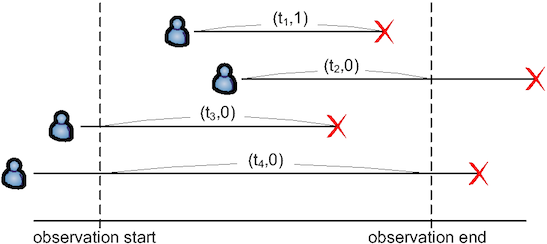Some software packages instead assume we have a censoring indicator: $c_i=\left\{\begin{array}{c} 0 \mbox{ if } T_i\leq U_i\\ 1 \mbox{ if } T_i> U_i \end{array}\right.$

Right-censoring is the most common type of censoring assumption we will deal with in survival analysis.

### Left-censoring

Can only observe $$Y_i=\max(T_i, U_i)$$ and the failure indicators: $\delta_i=\left\{\begin{array}{c} 1 \mbox{ if } U_i\leq T_i\\ 0 \mbox{ if } U_i> T_i \end{array}\right.$ e.g. (Miller) study of age at which African children learn a task. Some already knew (left-censored), some learned during study (exact), some had not yet learned by end of study (right-censored).

### Interval censoring

Observe $$(L_i, R_i)$$ where $$T_i\in (L_i, R_i)$$.

## More definitions and notation

There are several equivalent ways to characterize the probability distribution of a survival random variable. Some of these are familiar; others are special to survival analysis. We will focus on the following terms:

• The density function $$f(t)$$
• The survivor function $$S(t)$$
• The hazard function $$\lambda(t)$$
• The cumulative hazard function $$\Lambda(t)$$

### Density function

• discrete; Suppose that $$T$$ takes values in $$a_1, a_2,\ldots$$. $f(t)=\mathsf{P}[T=t]=\left\{\begin{array}{l} f_j \mbox{ if } t=a_j,\,j=1,2,\ldots\\ 0 \mbox{ otherwise} \end{array}\right.$
• continuous $f(t)=\lim_{\Delta t\to 0_+}\frac{\mathsf{P}[t\leq T\leq t+\Delta t]}{\Delta t}$

### Survivorship function

Survivorship (or survivor or survival) function $$S(t)=\mathsf{P}[T\geq t]$$.

In other settings, the cumulative distribution function, $$F(t)=\mathsf{P}[T\leq t]$$, is of interest. In survival analysis, our interest tends to focus on the survival function, $$S(t)$$.

• discrete $S(t)=\sum_{a_j\geq t}f_j$
• continuous $S(t)=\int_t^{\infty}f(u)\mbox{d}u$

Remarks:

• From the definition of $$S(t)$$ for a continuous variable, $$S(t)=1-F(t)$$ as long as $$f(t)$$ is absolutely continuous
• For a discrete variable, we have to decide what to do if an event occurs exactly at time $$t$$; i.e., does that become part of $$F(t)$$ or $$S(t)$$?
• To get around this problem, several books define $$S(t)=Pr(T>t)$$, or else define $$F(t)=\mathsf{P}[T < t]$$ (e.g. Collett)

### Hazard function

Sometimes called an instantaneous failure rate, the force of mortality, or the age-specific failure rate.

• discrete $\lambda_j=\mathsf{P}[T=a_j|T\geq a_j]=\frac{f_j}{\sum_{k:\,a_k\geq a_j}f_k}$
• continuous $\lambda(t)=\lim_{\Delta t\to 0_+}\frac{\mathsf{P}[t\leq T\leq t+\Delta t|T\geq t]}{\Delta t}=\frac{f(t)}{S(t)}$

### Cumulative hazard function

• discrete $\Lambda_j=\sum_{j:\,a_j\le t}\lambda_j$
• continuous $\Lambda(t)=\int_0^t\lambda(u)\mbox{d}u$

## Several relationships

$$S(t)$$ and $$\lambda(t)$$ for a continuous r. v. $\lambda(t)=-\frac{\mbox{d}}{\mbox{d}t}[\log S(t)]$

$$S(t)$$ and $$\Lambda(t)$$ for a continuous r. v. $S(t)=\exp\{-\Lambda(t)\}$

$$S(t)$$ and $$\Lambda(t)$$ for a discrete r. v. (suppose that $$a_j < t \leq a_{j+1}$$) $S(t)=\prod_{j:\,a_j < t}(1-\lambda_j)$ Cox defines $$\Lambda(t)=\sum_{j:\,a_j < t}\log (1-\lambda_j)$$ so that $$S(t)=\exp\{-\Lambda(t)\}$$ in the discrete case, as well.

## Measuring central tendency in survival

Mean survival - call this $$\mu$$

• discrete $\mu=\sum_j a_j f_j$
• continuous $\mu=\int_0^{\infty} uf(u)\mbox{d}u$

Median survival - call this $$\tau$$, is defined by $S(\tau)=0.5$ Similarly, any other percentile could be defined.

In practice, we don't usually hit the median survival at exactly one of the failure times. In this case, the estimated median survival is the smallest time $$\tau$$ such that $$S(\tau)\leq 0.5$$

# Estimating the survival or hazard function

We can estimate the survival (or hazard) function in two ways:

• by specifying a parametric model for $$\lambda(t)$$ based on a particular density function $$f(t)$$
• by developing an empirical estimate of the survival function (i.e., non-parametric estimation)

## If no censoring

The empirical estimate of the survival function, $$\widehat{S}(t)$$, is the proportion of individuals with event times greater than $$t$$.

## With censoring

If there are censored observations, then $$\widehat{S}(t)$$ is not a good estimate of the true $$S(t)$$, so other non-parametric methods must be used to account for censoring (life-table methods, Kaplan-Meier estimator)

## Preview of coming attractions

Next we will discuss the most famous non-parametric approach for estimating the survival distribution, called the Kaplan-Meier estimator.

To motivate the derivation of this estimator, we will first consider a set of survival times where there is no censoring.

The following are times to relapse (weeks) for 21 leukemia patients receiving control treatment (Table 1.1 of Cox & Oakes):

1, 1, 2, 2, 3, 4, 4, 5, 5, 8, 8, 8, 8, 11, 11, 12, 12, 15, 17, 22, 23

How would we estimate $$S(10)$$, the probability that an individual survives to time 10 or later?

What about $$\widehat{S}(8)$$? Is it $$12/21$$ or $$8/21$$?

## Empirical survival function

When there is no censoring, the general formula is: $\widehat{S}(t)=\frac{\# \mbox{ of individuals with } T\geq t}{\mbox{total sample size}}$ In most software packages, the survival function is evaluated just after time $$t$$, i.e., at $$t^+$$. In this case, we only count the individuals with $$T > t$$.

# Kaplan-Meier estimator

What if there is censoring? [Note: times with $$+$$ are right censored.]

6+, 6, 6, 6, 7, 9+, 10+, 10, 11+, 13, 16, 17+, 19+, 20+, 22, 23, 25+, 32+, 32+, 34+, 35+

We know $$\widehat{S}(6)=21/21$$, because everyone survived at least until time 6 or greater. But, we can't say $$\widehat{S}(7)=17/21$$, because we don't know the status of the person who was censored at time 6.

In a 1958 paper in the Journal of the American Statistical Association, Kaplan and Meier proposed a way to nonparametrically estimate $$S(t)$$, even in the presence of censoring. The method is based on the ideas of conditional probability.

Suppose $$a_k < t \leq a_{k+1}$$. Then $S(t)=\mathsf{P}[T\geq a_{k+1}]=\mathsf{P}[T\geq a_1,\ldots,T\geq a_{k+1}]=\prod_{j=1}^{k}\{1-\mathsf{P}[T=a_j|T\geq a_j]\}=\prod_{j=1}^{k}\{1-\lambda_j\}$ So $\widehat{S}(t)=\prod_j^{ a_j < t }\left(1-\frac{r_j}{d_j}\right)$ where $$d_j$$ is the number of deaths at $$a_j$$ and $$r_j$$ is the number at risk at $$a_j$$.

## Intuition behind the Kaplan-Meier estimator

Think of dividing the observed timespan of the study into a series of fine intervals so that there is a separate interval for each time of death or censoring:

_ , _ , D , _ , C , _ , C , D , D , D

Using the law of conditional probability, $\mathsf{P}[T\geq t]=\prod_j\mathsf{P}[\mbox{survive j th interval } I_j | \mbox{ survived to start of } I_j]$ where the product is taken over all the intervals including or preceding time $$t$$.

4 possibilities for each interval:

1. No events (death or censoring) - conditional probability of surviving the interval is 1
2. Censoring - assume they survive to the end of the interval, so that the conditional probability of surviving the interval is 1
3. Death, but no censoring - conditional probability of not surviving the interval is # deaths ($$d$$) divided by # 'at risk' ($$r$$) at the beginning of the interval. So the conditional probability of surviving the interval is $$1 - (d/r)$$
4. Tied deaths and censoring - assume censorings last to the end of the interval, so that conditional probability of surviving the interval is still $$1 - (d/r)$$

### General Formula for jth interval

It turns out we can write a general formula for the conditional probability of surviving the j-th interval that holds for all 4 cases.

We could use the same approach by grouping the event times into intervals (say, one interval for each month), and then counting up the number of deaths (events) in each to estimate the probability of surviving the interval (this is called the lifetable estimate).

However, the assumption that those censored last until the end of the interval wouldn't be quite accurate, so we would end up with a cruder approximation.

As the intervals get finer and finer, the approximations made in estimating the probabilities of getting through each interval become smaller and smaller, so that the estimator converges to the true $$S(t)$$.

This intuition clarifies why an alternative name for the KM is the product limit estimator.

## KM estimator

$\widehat{S}(t)=\prod_j^{ \tau_j < t }\left(1-\frac{r_j}{d_j}\right)$ where

• $$\tau_1,\ldots,\tau_K$$ is the set of $$K$$ distinct death times observed in the sample
• $$d_j$$ is the number of deaths at $$\tau_j$$
• $$r_j$$ is the number of individuals 'at risk' right before the j-th death time (everyone dead or censored at or after that time).
• $$c_j$$ is the number of censored observations between the j-th and (j+1)-st death times. Censorings tied at $$\tau_j$$ are included in $$c_j$$

Remarks:

• $$r_j=r_{j-1}-d_{j-1}-c_{j-1}$$
• $$r_j=\sum_{l\geq j}(c_l+d_l)$$
• $$\widehat{S}(t^+)$$ changes only ath the death (failure) times
• $$\widehat{S}(t^+)$$ is 1 up to the first death time
• $$\widehat{S}(t^+)$$ only goes to 0 if the last event is a death

## Leukemia data set

The file leukAB.dat contains the failure/censoring times and the event indicators for the Leukemia example (treatment arm).

# read dataset, skip 2 lines of file:
leuk = read.table("http://www.karlin.mff.cuni.cz/~pesta/prednasky/NMFM404/Data/leukAB.dat",
sep=" ", head=T, skip=4)
# print a few observations; t = time, f = event indicator
leuk[1:3,]

##   t f
## 1 6 0
## 2 6 1
## 3 6 1

fit.leuk = survfit(Surv(leuk$t, leuk$f) ~ 1)
summary(fit.leuk)

## Call: survfit(formula = Surv(leuk$t, leuk$f) ~ 1)
##
##  time n.risk n.event survival std.err lower 95% CI upper 95% CI
##     6     21       3    0.857  0.0764        0.720        1.000
##     7     17       1    0.807  0.0869        0.653        0.996
##    10     15       1    0.753  0.0963        0.586        0.968
##    13     12       1    0.690  0.1068        0.510        0.935
##    16     11       1    0.627  0.1141        0.439        0.896
##    22      7       1    0.538  0.1282        0.337        0.858
##    23      6       1    0.448  0.1346        0.249        0.807


plot(fit.leuk, xlab="Weeks", ylab="Proportion Survivors", col=3)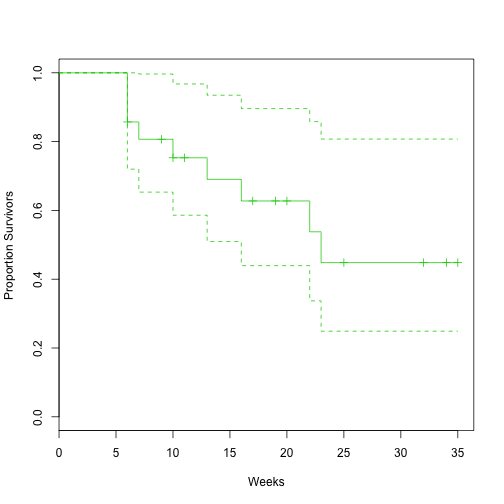## Confidence intervals

### Greenwood's formula

Variance of KM: $\mathsf{var}\widehat{S}(t)=\left[\widehat{S}(t)\right]^2\sum_j^{ \tau_j < t }\frac{d_j}{r_j(d_j-r_j)}$ For a 95% confidence interval, we could use $\widehat{S}(t)\pm z_{1-\alpha/2}\mathsf{se}\left(\widehat{S}(t)\right)$ where $$\mathsf{se}\left(\widehat{S}(t)\right)$$ is calculated using Greenwood's formula.

### Log-log approach

Problem: This approach can yield values $$> 1$$ or $$< 0$$.

Better approach: Get a 95% confidence interval for $$L(t) = \log(- \log(S(t)))$$, i.e., log-log approach. Since this quantity is unrestricted, the confidence interval will be in the proper range when we transform back.

Applying the delta method, we get: $\mathsf{var}\widehat{L}(t)=\frac{1}{\left[\log \widehat{S}(t)\right]^2}\sum_j^{ \tau_j < t }\frac{d_j}{r_j(d_j-r_j)}$ We take the square root of the above to get $$\mathsf{se}\left(\widehat{L}(t)\right)$$, and then form the confidence intervals as: $\widehat{S}(t)^{\exp\left\{\pm 1.96\mathsf{se}\left(\widehat{L}(t)\right)\right\}}$ R gives an option to calculate these bounds (use conf.type='log-log' in survfit).

### Log stabilizing transformation

R (by default) uses a log transformation to stabilize the variance and allow for non-symmetric confidence intervals. This is what is normally done for the confidence interval of an estimated odds ratio. $\mathsf{var}\left(\log \widehat{L}(t)\right)=\sum_j^{ \tau_j < t }\frac{d_j}{r_j(d_j-r_j)}$

summary(fit.leuk)     # CI = "log"

## Call: survfit(formula = Surv(leuk$t, leuk$f) ~ 1)
##
##  time n.risk n.event survival std.err lower 95% CI upper 95% CI
##     6     21       3    0.857  0.0764        0.720        1.000
##     7     17       1    0.807  0.0869        0.653        0.996
##    10     15       1    0.753  0.0963        0.586        0.968
##    13     12       1    0.690  0.1068        0.510        0.935
##    16     11       1    0.627  0.1141        0.439        0.896
##    22      7       1    0.538  0.1282        0.337        0.858
##    23      6       1    0.448  0.1346        0.249        0.807

fit.leuk2 = survfit(Surv(leuk$t, leuk$f) ~ 1, conf.type="log-log")
summary(fit.leuk2)

## Call: survfit(formula = Surv(leuk$t, leuk$f) ~ 1, conf.type = "log-log")
##
##  time n.risk n.event survival std.err lower 95% CI upper 95% CI
##     6     21       3    0.857  0.0764        0.620        0.952
##     7     17       1    0.807  0.0869        0.563        0.923
##    10     15       1    0.753  0.0963        0.503        0.889
##    13     12       1    0.690  0.1068        0.432        0.849
##    16     11       1    0.627  0.1141        0.368        0.805
##    22      7       1    0.538  0.1282        0.268        0.747
##    23      6       1    0.448  0.1346        0.188        0.680

fit.leuk3 = survfit(Surv(leuk$t, leuk$f) ~ 1, conf.type="plain")
summary(fit.leuk3)        # notice CI symmetrical about S_hat

## Call: survfit(formula = Surv(leuk$t, leuk$f) ~ 1, conf.type = "plain")
##
##  time n.risk n.event survival std.err lower 95% CI upper 95% CI
##     6     21       3    0.857  0.0764        0.707        1.000
##     7     17       1    0.807  0.0869        0.636        0.977
##    10     15       1    0.753  0.0963        0.564        0.942
##    13     12       1    0.690  0.1068        0.481        0.900
##    16     11       1    0.627  0.1141        0.404        0.851
##    22      7       1    0.538  0.1282        0.286        0.789
##    23      6       1    0.448  0.1346        0.184        0.712


### ggplot2 for KM

Using ggplot2 ggsurv function for KM estimnator.

ggsurv <- function(s, CI = 'def', plot.cens = T, surv.col = 'gg.def',
cens.col = 'red', lty.est = 1, lty.ci = 2,
cens.shape = 3, back.white = F, xlab = 'Time',
ylab = 'Survival', main = ''){

library(ggplot2)
strata <- ifelse(is.null(s$strata) ==T, 1, length(s$strata))
stopifnot(length(surv.col) == 1 | length(surv.col) == strata)
stopifnot(length(lty.est) == 1 | length(lty.est) == strata)

ggsurv.s <- function(s, CI = 'def', plot.cens = T, surv.col = 'gg.def',
cens.col = 'red', lty.est = 1, lty.ci = 2,
cens.shape = 3, back.white = F, xlab = 'Time',
ylab = 'Survival', main = ''){

dat <- data.frame(time = c(0, s$time), surv = c(1, s$surv),
up = c(1, s$upper), low = c(1, s$lower),
cens = c(0, s$n.censor)) dat.cens <- subset(dat, cens != 0) col <- ifelse(surv.col == 'gg.def', 'black', surv.col) pl <- ggplot(dat, aes(x = time, y = surv)) + xlab(xlab) + ylab(ylab) + ggtitle(main) + geom_step(col = col, lty = lty.est) pl <- if(CI == T | CI == 'def') { pl + geom_step(aes(y = up), color = col, lty = lty.ci) + geom_step(aes(y = low), color = col, lty = lty.ci) } else (pl) pl <- if(plot.cens == T & length(dat.cens) > 0){ pl + geom_point(data = dat.cens, aes(y = surv), shape = cens.shape, col = cens.col) } else if (plot.cens == T & length(dat.cens) == 0){ stop ('There are no censored observations') } else(pl) pl <- if(back.white == T) {pl + theme_bw() } else (pl) pl } ggsurv.m <- function(s, CI = 'def', plot.cens = T, surv.col = 'gg.def', cens.col = 'red', lty.est = 1, lty.ci = 2, cens.shape = 3, back.white = F, xlab = 'Time', ylab = 'Survival', main = '') { n <- s$strata

groups <- factor(unlist(strsplit(names
(s$strata), '='))[seq(2, 2*strata, by = 2)]) gr.name <- unlist(strsplit(names(s$strata), '='))
gr.df <- vector('list', strata)
ind <- vector('list', strata)
n.ind <- c(0,n); n.ind <- cumsum(n.ind)
for(i in 1:strata) ind[[i]] <- (n.ind[i]+1):n.ind[i+1]

for(i in 1:strata){
gr.df[[i]] <- data.frame(
time = c(0, s$time[ ind[[i]] ]), surv = c(1, s$surv[ ind[[i]] ]),
up = c(1, s$upper[ ind[[i]] ]), low = c(1, s$lower[ ind[[i]] ]),
cens = c(0, s$n.censor[ ind[[i]] ]), group = rep(groups[i], n[i] + 1)) } dat <- do.call(rbind, gr.df) dat.cens <- subset(dat, cens != 0) pl <- ggplot(dat, aes(x = time, y = surv, group = group)) + xlab(xlab) + ylab(ylab) + ggtitle(main) + geom_step(aes(col = group, lty = group)) col <- if(length(surv.col == 1)){ scale_colour_manual(name = gr.name, values = rep(surv.col, strata)) } else{ scale_colour_manual(name = gr.name, values = surv.col) } pl <- if(surv.col != 'gg.def'){ pl + col } else {pl + scale_colour_discrete(name = gr.name)} line <- if(length(lty.est) == 1){ scale_linetype_manual(name = gr.name, values = rep(lty.est, strata)) } else {scale_linetype_manual(name = gr.name, values = lty.est)} pl <- pl + line pl <- if(CI == T) { if(length(surv.col) > 1 && length(lty.est) > 1){ stop('Either surv.col or lty.est should be of length 1 in order to plot 95% CI with multiple strata') }else if((length(surv.col) > 1 | surv.col == 'gg.def')){ pl + geom_step(aes(y = up, color = group), lty = lty.ci) + geom_step(aes(y = low, color = group), lty = lty.ci) } else{pl + geom_step(aes(y = up, lty = group), col = surv.col) + geom_step(aes(y = low,lty = group), col = surv.col)} } else {pl} pl <- if(plot.cens == T & length(dat.cens) > 0){ pl + geom_point(data = dat.cens, aes(y = surv), shape = cens.shape, col = cens.col) } else if (plot.cens == T & length(dat.cens) == 0){ stop ('There are no censored observations') } else(pl) pl <- if(back.white == T) {pl + theme_bw() } else (pl) pl } pl <- if(strata == 1) {ggsurv.s(s, CI , plot.cens, surv.col , cens.col, lty.est, lty.ci, cens.shape, back.white, xlab, ylab, main) } else {ggsurv.m(s, CI, plot.cens, surv.col , cens.col, lty.est, lty.ci, cens.shape, back.white, xlab, ylab, main)} pl }  ggsurv(fit.leuk)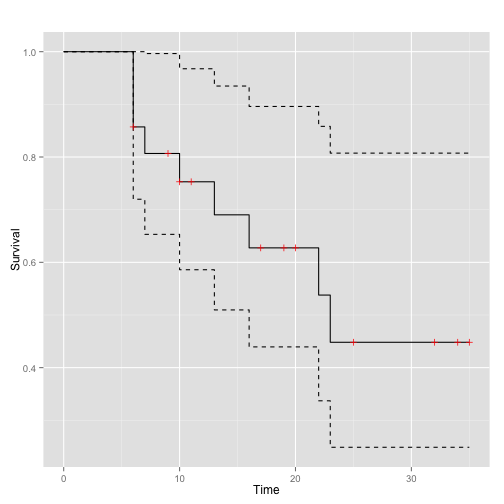# Lifetable or actuarial estimator Applies when the data are grouped. Our goal is still to estimate the survival function, hazard, and density function, but this is complicated by the fact that we don't know exactly when during each time interval an event occurs. Notation: • the j-th time interval is $$[t_{j-1},t_j)$$ • $$c_j$$ - the number of censorings in the j-th interval • $$d_j$$ - the number of failures in the j-th interval • $$r_j$$ - the number entering the interval We could apply the K-M formula directly. However, this approach is unsatisfactory for grouped data ... it treats the problem as though it were in discrete time, with events happening only at 1 yr, 2 yr, etc. In fact, what we are trying to calculate here is the conditional probability of dying within the interval, given survival to the beginning of it. We can assume that censorings occur on average halfway through the interval: $r_j'=r_j-c_j/2$ The assumption yields the Actuarial Estimator. It is appropriate if censorings occur uniformly throughout the interval. Remarks: • Because the intervals are defined as $$[t_{j-1}, t_j)$$, the first interval typically starts with $$t_0 = 0$$. • R and SAS estimate the survival function at the left-hand endpoint, $$S(t_{j-1})$$. Stata at the right-hand one. • The implication in R and SAS is that $$\widehat{S}(t_0)=1$$. ## Quantities estimated • Midpoint $$t_{mj}=(t_j+t_{j-1})/2$$ • Width $$b_j=t_j-t_{j-1}$$ • Conditional probability of dying $$\widehat{q}_j=d_j/r_j'$$ • Conditional probability of surviving $$\widehat{p}_j=1-\widehat{q}_j$$ • Cumulative probability of surviving at $$t_j$$: $$\widehat{S}(t)=\prod_{l\leq j}\widehat{p}_l$$ • Hazard in the j-th interval $\widehat{\lambda}(t_{mj})=\frac{\widehat{q}_j}{b_j(1-\widehat{q}_j/2)}$ the number of deaths in the interval divided by the average number of survivors at the midpoint • Density at the midpoint of the j-th interval $\widehat{f}(t_{mj})=\frac{\widehat{S}(t_{j-1})\widehat{q}_j}{b_j}$ ### Constructing the lifetable R with KMsurv package requires three elements: • a vector of $$k + 1$$ interval endpoints • a $$k$$ vector of death counts in each interval • a $$k$$ vector of censored counts in each interval Incidentally, KM in KMsurv stands for Klein and Moeschberger, not Kaplan-Meier! ### Angina Pectoris data intEndpts = 0:16 # interval endpoints deaths = c(456, 226, 152, 171, 135, 125, 83, 74, 51, 42, 43, 34, 18, 9, 6, 0) cens = c(0, 39, 22, 23, 24, 107, 133, 102, 68, 64, 45, 53, 33, 27, 23, 30) # n censored fitlt = lifetab(tis = intEndpts, ninit=2418, nlost=cens, nevent=deaths) round(fitlt, 4) # restrict output to 4 decimals  ## nsubs nlost nrisk nevent surv pdf hazard se.surv se.pdf ## 0-1 2418 0 2418.0 456 1.0000 0.1886 0.2082 0.0000 0.0080 ## 1-2 1962 39 1942.5 226 0.8114 0.0944 0.1235 0.0080 0.0060 ## 2-3 1697 22 1686.0 152 0.7170 0.0646 0.0944 0.0092 0.0051 ## 3-4 1523 23 1511.5 171 0.6524 0.0738 0.1199 0.0097 0.0054 ## 4-5 1329 24 1317.0 135 0.5786 0.0593 0.1080 0.0101 0.0049 ## 5-6 1170 107 1116.5 125 0.5193 0.0581 0.1186 0.0103 0.0050 ## 6-7 938 133 871.5 83 0.4611 0.0439 0.1000 0.0104 0.0047 ## 7-8 722 102 671.0 74 0.4172 0.0460 0.1167 0.0105 0.0052 ## 8-9 546 68 512.0 51 0.3712 0.0370 0.1048 0.0106 0.0050 ## 9-10 427 64 395.0 42 0.3342 0.0355 0.1123 0.0107 0.0053 ## 10-11 321 45 298.5 43 0.2987 0.0430 0.1552 0.0109 0.0063 ## 11-12 233 53 206.5 34 0.2557 0.0421 0.1794 0.0111 0.0068 ## 12-13 146 33 129.5 18 0.2136 0.0297 0.1494 0.0114 0.0067 ## 13-14 95 27 81.5 9 0.1839 0.0203 0.1169 0.0118 0.0065 ## 14-15 59 23 47.5 6 0.1636 0.0207 0.1348 0.0123 0.0080 ## 15-16 30 30 15.0 0 0.1429 NA NA 0.0133 NA ## se.hazard ## 0-1 0.0097 ## 1-2 0.0082 ## 2-3 0.0076 ## 3-4 0.0092 ## 4-5 0.0093 ## 5-6 0.0106 ## 6-7 0.0110 ## 7-8 0.0135 ## 8-9 0.0147 ## 9-10 0.0173 ## 10-11 0.0236 ## 11-12 0.0306 ## 12-13 0.0351 ## 13-14 0.0389 ## 14-15 0.0549 ## 15-16 NA  ### Duration of nursing home stay data The National Center for Health Services Research studied 36 for-profit nursing homes to assess the effects of different financial incentives on length of stay. 'Treated' nursing homes received higher per diems for Medicaid patients, and bonuses for improving a patient's health and sending them home. Study included 1601 patients admitted between May 1, 1981 and April 30, 1982. Variables include: • los - Length of stay of a resident (in days) • age - Age of a resident • rx - Nursing home assignment (1:bonuses, 0:no bonuses) • gender - Gender (1:male, 0:female) • married - (1:married, 0:not married) • health - Health status (2:second best, 5:worst) • censor - Censoring indicator (1:censored, 0:discharged) Suppose we wish to use the actuarial method, but the data do not come grouped. Consider the treated nursing home patients, with length of stay (los) grouped into 100 day intervals: nurshome = read.table("http://www.karlin.mff.cuni.cz/~pesta/prednasky/NMFM404/Data/NursingHome.dat", head=F, skip=14, col.names = c("los", "age", "rx", "gender", "married", "health", "censor")) nurshome$int = floor(nurshome$los/100)*100 # group in 100 day intervals nurshome = nurshome[nurshome$rx==1,]   # keep only treated homes
nurshome[1:3,]    # check out a few observations

##    los age rx gender married health censor  int
## 1   37  86  1      0       0      2      0    0
## 2   61  77  1      0       0      4      0    0
## 3 1084  75  1      0       0      3      1 1000

tab = table(nurshome$int, nurshome$censor)
intEndpts = (0:11)*100
ntotal = dim(nurshome)  # nr patients
cens = tab[,2]   # nr censored in each interval
released = tab[,1]   # nr released in each interval
fitlt = lifetab(tis = intEndpts, ninit=ntotal, nlost=cens, nevent=released)
round(fitlt, 4)        # restrict output to 4 decimals

##           nsubs nlost nrisk nevent   surv    pdf hazard se.surv se.pdf
## 0-100       712     0 712.0    330 1.0000 0.0046 0.0060  0.0000  2e-04
## 100-200     382     0 382.0     86 0.5365 0.0012 0.0025  0.0187  1e-04
## 200-300     296     0 296.0     65 0.4157 0.0009 0.0025  0.0185  1e-04
## 300-400     231     0 231.0     38 0.3244 0.0005 0.0018  0.0175  1e-04
## 400-500     193     1 192.5     32 0.2711 0.0005 0.0018  0.0167  1e-04
## 500-600     160     0 160.0     13 0.2260 0.0002 0.0008  0.0157  1e-04
## 600-700     147     0 147.0     13 0.2076 0.0002 0.0009  0.0152  1e-04
## 700-800     134    30 119.0     10 0.1893 0.0002 0.0009  0.0147  0e+00
## 800-900      94    29  79.5      4 0.1734 0.0001 0.0005  0.0143  0e+00
## 900-1000     61    30  46.0      4 0.1647 0.0001 0.0009  0.0142  1e-04
## 1000-1100    27    27  13.5      0 0.1503     NA     NA  0.0147     NA
##           se.hazard
## 0-100         3e-04
## 100-200       3e-04
## 200-300       3e-04
## 300-400       3e-04
## 400-500       3e-04
## 500-600       2e-04
## 600-700       3e-04
## 700-800       3e-04
## 800-900       3e-04
## 900-1000      5e-04
## 1000-1100        NA


Estimated Survival

names(fitlt)  # check out components of fitlt object

##   "nsubs"     "nlost"     "nrisk"     "nevent"    "surv"
##   "pdf"       "hazard"    "se.surv"   "se.pdf"    "se.hazard"

x = rep(intEndpts, rep(2,12))[2:23]
y = rep(fitlt$surv, rep(2,11))  plot(x, y, type="l", col=4, xlab="Time Interval (Days)", ylab="Survival (Life Table)") title(main = "Duration of stay in nursing homes", cex=.6)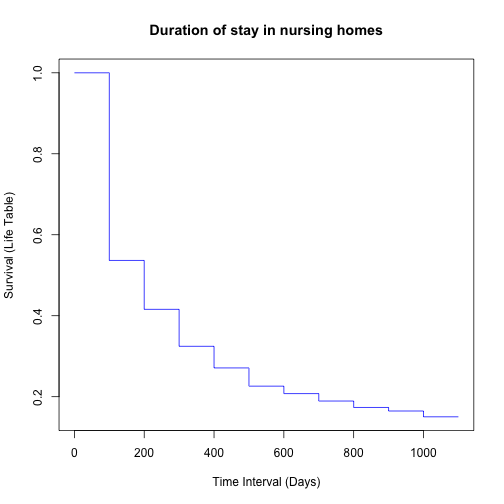Estimated Hazard y = rep(fitlt$hazard, rep(2,11))


plot(x, y, type="l", col=6, xlab="Time Interval (Days)", ylab="Hazard (Life Table)")
title(main = "Duration of stay in nursing homes", cex=.6)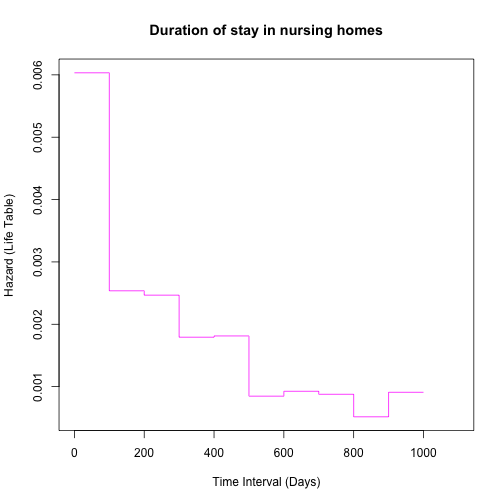# Nelson-Aalen estimator

Estimating the cumulative hazard

$$\Lambda(t)$$ can then be approximated by a sum: $\widehat{\Lambda}(t)=\sum_j\lambda_j\Delta$ where the sum is over intervals, $$\lambda_j$$ is the value of the hazard in the j-th interval and $$\Delta$$ is the width of each interval. Since $$\lambda_j\Delta$$ is approximately the probability of dying in the interval, we can further approximate by $\widehat{\Lambda}(t)=\sum_j\frac{d_j}{r_j}$ It follows that $$\Lambda(t)$$ will change only at death times, and hence we write the Nelson-Aalen estimator as: $\widehat{\Lambda}_{NA}(t)=\sum_{j}^{ \tau_j < t }\frac{d_j}{r_j}$

Once we have $$\widehat{\Lambda}_{NA}(t)$$, we can also find another estimator of $$S(t)$$ (Fleming-Harrington): $\widehat{S}_{FM}(t)=\exp\{-\widehat{\Lambda}_{NA}(t)\}$

Say we want to obtain the Fleming-Harrington estimate of the survival function for married females, in the healthiest initial subgroup, who are randomized to the untreated group of the nursing home study.

nurshome = read.table("http://www.karlin.mff.cuni.cz/~pesta/prednasky/NMFM404/Data/NursingHome.dat",
head=F, skip=14, col.names = c("los", "age", "rx", "gender", "married", "health", "censor"))
(nurs2 = subset(nurshome, gender==0 & rx==0 & health==2 & married==1))

##      los age rx gender married health censor
## 144   89  78  0      0       1      2      0
## 362  123  75  0      0       1      2      0
## 427   38  78  0      0       1      2      0
## 601   24  82  0      0       1      2      0
## 719  185  86  0      0       1      2      0
## 736  113  73  0      0       1      2      0
## 1120  25  71  0      0       1      2      0
## 1343  14  73  0      0       1      2      0
## 1362 149  81  0      0       1      2      0
## 1461 168  72  0      0       1      2      0
## 1472  64  81  0      0       1      2      0
## 1489 234  72  0      0       1      2      0

fit.fh = survfit(Surv(nurs2$los, 1-nurs2$censor) ~ 1,
type="fleming-harrington", conf.type="log-log")
summary(fit.fh)

## Call: survfit(formula = Surv(nurs2$los, 1 - nurs2$censor) ~ 1, type = "fleming-harrington",
##     conf.type = "log-log")
##
##  time n.risk n.event survival std.err lower 95% CI upper 95% CI
##    14     12       1   0.9200  0.0801       0.5244        0.989
##    24     11       1   0.8401  0.1085       0.4750        0.960
##    25     10       1   0.7601  0.1267       0.4056        0.920
##    38      9       1   0.6802  0.1388       0.3368        0.872
##    64      8       1   0.6003  0.1465       0.2718        0.819
##    89      7       1   0.5204  0.1502       0.2116        0.760
##   113      6       1   0.4405  0.1505       0.1564        0.696
##   123      5       1   0.3606  0.1472       0.1070        0.628
##   149      4       1   0.2809  0.1404       0.0641        0.556
##   168      3       1   0.2012  0.1299       0.0293        0.483
##   185      2       1   0.1221  0.1169       0.0059        0.422
##   234      1       1   0.0449     Inf       0.0000        1.000

# compare with KM:
fit.km = survfit(Surv(nurs2$los, 1-nurs2$censor) ~ 1,
type="kaplan-meier", conf.type="log-log")
summary(fit.km)

## Call: survfit(formula = Surv(nurs2$los, 1 - nurs2$censor) ~ 1, type = "kaplan-meier",
##     conf.type = "log-log")
##
##  time n.risk n.event survival std.err lower 95% CI upper 95% CI
##    14     12       1   0.9167  0.0798      0.53898        0.988
##    24     11       1   0.8333  0.1076      0.48171        0.956
##    25     10       1   0.7500  0.1250      0.40842        0.912
##    38      9       1   0.6667  0.1361      0.33702        0.860
##    64      8       1   0.5833  0.1423      0.27014        0.801
##    89      7       1   0.5000  0.1443      0.20848        0.736
##   113      6       1   0.4167  0.1423      0.15247        0.665
##   123      5       1   0.3333  0.1361      0.10270        0.588
##   149      4       1   0.2500  0.1250      0.06014        0.505
##   168      3       1   0.1667  0.1076      0.02651        0.413
##   185      2       1   0.0833  0.0798      0.00505        0.311
##   234      1       1   0.0000     NaN           NA           NA


# Comparison of survival curves

## Two survival curves

\begin{align*} H_0&:\,S_1(t)=S_2(t),\,\forall t\\ H_1&:\,\exists t:\,S_1(t)\neq S_2(t) \end{align*}

## Cochran-Mantel-Haenszel Logrank test

The logrank test is the most well known and widely used.

It also has an intuitive appeal, building on standard methods for binary data. (Later we will see that it can also be obtained as the score test from a partial likelihood from the Cox Proportional Hazards model.)

The logrank test is obtained by constructing a 2x2 table at each distinct death time, and comparing the death rates between the two groups, conditional on the number at risk in the groups. The tables are then combined using the Cochran-Mantel-Haenszel test. Note that the logrank is sometimes called the Cox-Mantel test.

leuk = read.table("http://www.karlin.mff.cuni.cz/~pesta/prednasky/NMFM404/Data/leuk.dat",
sep=" ", head=T, skip=7)
summary(leuk)

##      weeks           remiss           trtmt
##  Min.   : 1.00   Min.   :0.0000   Min.   :0.0
##  1st Qu.: 6.00   1st Qu.:0.0000   1st Qu.:0.0
##  Median :10.50   Median :1.0000   Median :0.5
##  Mean   :12.88   Mean   :0.7143   Mean   :0.5
##  3rd Qu.:18.50   3rd Qu.:1.0000   3rd Qu.:1.0
##  Max.   :35.00   Max.   :1.0000   Max.   :1.0

## Log Rank test
(leuk.lrt = survdiff(Surv(weeks,remiss) ~ trtmt, data = leuk))

## Call:
## survdiff(formula = Surv(weeks, remiss) ~ trtmt, data = leuk)
##
##          N Observed Expected (O-E)^2/E (O-E)^2/V
## trtmt=0 21       21     10.7      9.77      16.8
## trtmt=1 21        9     19.3      5.46      16.8
##
##  Chisq= 16.8  on 1 degrees of freedom, p= 4.17e-05


• The logrank statistic depends on ranks of event times only.
• It does not matter which group you choose.
• Analogous to the CMH test for a series of tables at different levels of a confounder, the logrank test is most powerful when 'odds ratios' are constant over time intervals. That is, it is most powerful for proportional hazards.

## Peto-Peto logrank test

Logrank test as a linear rank test

The logrank test can be derived by assigning scores to the ranks of the death times. This is called the Peto-Peto logrank test, or the linear rank logrank test.

### Comparing the CMH-type Logrank and Peto-Peto Logrank

• CMH-type Logrank: We motivated the logrank test through the CMH statistic for testing $$H_0:\,OR=1$$ over $$K$$ tables, where $$K$$ is the number of distinct death times.
• Peto-Peto (linear rank) Logrank: The linear rank version of the logrank test is based on adding up 'scores' for one of the two treatment groups. The particular scores that gave us the same logrank statistic were based on the Nelson-Aalen estimator.
• If there are no tied event times, then the two versions of the test will yield identical results. The more ties we have, the more it matters which version we use.
• The Peto-Peto (linear rank) logrank test is not available in R. Use the CMH logrank test instead.

## Peto-Peto-Prentice-Gehan-Wilcoxon test

This is the Prentice (1978) modification of the Peto & Peto (1972) modification of the Gehan (1965) modification of the Wilcoxon (1945) rank test.

## Peto-Peto-Prentice-Gehan-Wilcoxon Rank test
## notice: rho=1
(leuk.pppgw = survdiff(Surv(weeks,remiss) ~ trtmt, data = leuk, rho=1))

## Call:
## survdiff(formula = Surv(weeks, remiss) ~ trtmt, data = leuk,
##     rho = 1)
##
##          N Observed Expected (O-E)^2/E (O-E)^2/V
## trtmt=0 21    14.55     7.68      6.16      14.5
## trtmt=1 21     5.12    12.00      3.94      14.5
##
##  Chisq= 14.5  on 1 degrees of freedom, p= 0.000143


leuk.km = survfit(Surv(weeks, remiss) ~ trtmt, data=leuk)


plot(leuk.km, xlab = "Weeks", ylab = "Survival", col=c(2,3))
legend(18, .95, legend=c("No treatment", "Treatment"),
col=c(2:3), lty=1)
title(main="Treatment Comparison, Leukemia Data", cex=.7)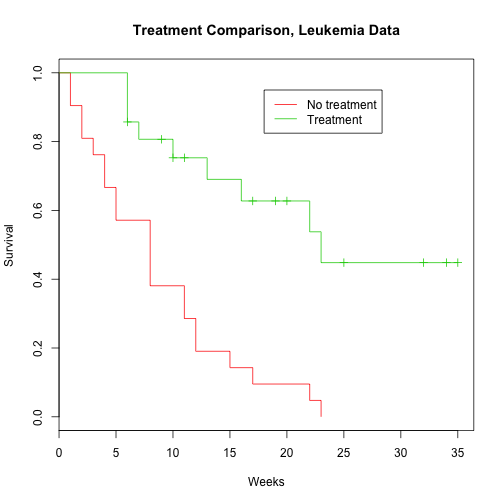(ggkm <- ggsurv(leuk.km))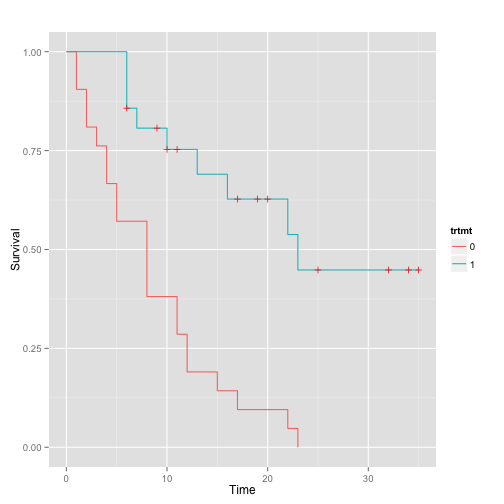### Which test should we use?

• Logrank test: CMH or Linear Rank? If there are not too many ties, then it doesn't really matter.
• Logrank test or (PPP)Gehan-Wilcoxon? This is a more important choice.
• Both tests have the correct level (Type I error) for testing the null hypothesis of equal survival, $$H_0:\,S_1(t)=S_2(t)$$.
• The choice of which test to use depends on the alternative hypothesis. This drives the power of the test.
• The Gehan-Wilcoxon test is sensitive to early differences in survival between groups.
• The Logrank test is sensitive to later differences.
• The logrank is most powerful under the assumption of proportional hazards, $$\lambda_1(t) = \alpha\lambda_2(t)$$, which implies an alternative in terms of the survival functions of $$H_1\, :S_1(t) = [S_2(t)]^{\alpha}$$.
• The Wilcoxon has high power when the failure times are lognormally distributed, with equal variance in both groups but a different mean. It will turn out that this is the assumption of an accelerated failure time model.
• Both tests will lack power if the survival curves (or hazards) 'cross' (not proportional hazards). However, that does not necessarily make them invalid!

## Three or more survival curves - $$P$$-sample logrank

There are more than two groups, and the question of interest is whether the groups differ from each other.

Example: Time taken to finish a test with 3 different noise distractions. All tests were stopped after 12 minutes.

time = c(9, 9.5, 9, 8.5, 10, 10.5, 10, 12, 12, 11, 12,
10.5, 12, 12, 12, 12, 12, 12)
event = c(1,1,1,1,1,1,1,1,0,1,1,1,1,0,0,0,0,0)
group = rep(1:3, times=c(6,6,6))
(cbind(group,time,event))

##       group time event
##  [1,]     1  9.0     1
##  [2,]     1  9.5     1
##  [3,]     1  9.0     1
##  [4,]     1  8.5     1
##  [5,]     1 10.0     1
##  [6,]     1 10.5     1
##  [7,]     2 10.0     1
##  [8,]     2 12.0     1
##  [9,]     2 12.0     0
## [10,]     2 11.0     1
## [11,]     2 12.0     1
## [12,]     2 10.5     1
## [13,]     3 12.0     1
## [14,]     3 12.0     0
## [15,]     3 12.0     0
## [16,]     3 12.0     0
## [17,]     3 12.0     0
## [18,]     3 12.0     0

(noise.lrt = survdiff(Surv(time, event) ~ group, rho=0))

## Call:
## survdiff(formula = Surv(time, event) ~ group, rho = 0)
##
##         N Observed Expected (O-E)^2/E (O-E)^2/V
## group=1 6        6     1.57   12.4463   17.2379
## group=2 6        5     4.53    0.0488    0.0876
## group=3 6        1     5.90    4.0660    9.4495
##
##  Chisq= 20.4  on 2 degrees of freedom, p= 3.75e-05


noise.km = survfit(Surv(time, event) ~ group)
plot(noise.km, col = 2:4, xlab = "Minutes",
ylab = "Time to finish test",
main = "Time to finish test for 3 noise levels")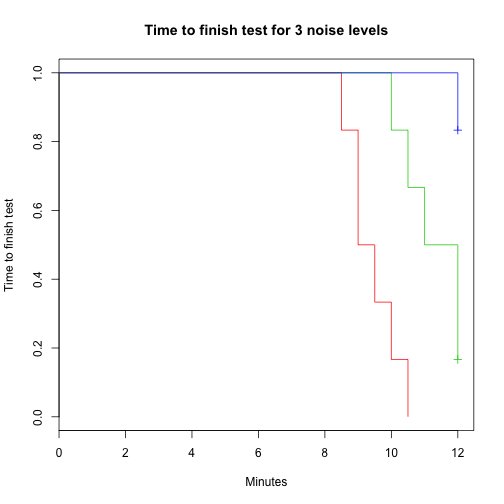## Stratified Logrank

Sometimes, even though we are interested in comparing two groups (or maybe $$P$$) groups, we know there are other factors that also affect the outcome. It would be useful to adjust for these other factors in some way.

Example: For the nursing home data, a logrank test comparing length of stay for those under and over 85 years of age suggests a significant difference ($$p=0.03$$). However, we know that gender has a strong association with length of stay, and also age. Hence, it would be a good idea to stratify the analysis by gender when trying to assess the age effect.

A stratified logrank allows one to compare groups, but allows the shapes of the hazards of the different groups to differ across strata. It makes the assumption that the group 1 vs group 2 hazard ratio is constant across strata. In other words: $$\lambda_{1s}(t)/\lambda_{2s}(t)=\theta$$ where $$\theta$$ is constant over the strata ($$s =1,\ldots,S$$).

nurshome =
read.table("http://www.karlin.mff.cuni.cz/~pesta/prednasky/NMFM404/Data/NursingHome.dat",
head=F, skip=14, col.names = c("los", "age", "rx", "gender", "married", "health", "censor"))
nurshome$discharged = 1 - nurshome$censor # event indicator
nurshome$under85 = (nurshome$age < 85) + 0
(nurs.slrt = survdiff( Surv(los, discharged) ~ under85 + strata(gender), data = nurshome))

## Call:
## survdiff(formula = Surv(los, discharged) ~ under85 + strata(gender),
##     data = nurshome)
##
##             N Observed Expected (O-E)^2/E (O-E)^2/V
## under85=0 689      537      560     0.926      1.73
## under85=1 912      742      719     0.721      1.73
##
##  Chisq= 1.7  on 1 degrees of freedom, p= 0.189


# References

• Collett: Modelling Survival Data in Medical Research
• Cox & Oakes: Analysis of Survival Data
• Kalbfleisch & Prentice: The Statistical Analysis of Failure Time Data
• Lee: Statistical Methods for Survival Data Analysis
• Fleming & Harrington: Counting Processes and Survival Analysis
• Hosmer & Lemeshow: Applied Survival Analysis
• Kleinbaum: Survival Analysis: A self-learning text
• Klein & Moeschberger: Survival Analysis: Techniques for censored and truncated data
• Cantor: Extending SAS Survival Analysis Techniques for Medical Research
• Allison: Survival Analysis Using the SAS System
• Jennison & Turnbull: Group Sequential Methods with Applications to Clinical Trials